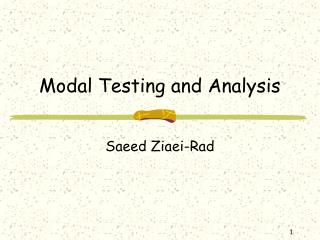DownloadDownload PresentationModal Testing and Analysis

# Modal Testing and Analysis

Télécharger la présentation## Modal Testing and Analysis

- - - - - - - - - - - - - - - - - - - - - - - - - - - E N D - - - - - - - - - - - - - - - - - - - - - - - - - - -
##### Presentation Transcript

1. Modal Testing and Analysis Saeed Ziaei-Rad

2. Single Degree-of-Freedom (SDOF) • Undamped • Viscously Damped • Hysterically (Structurally) Damped Modal Analysis and Testing

3. Undamped Systems (Theory) F(t) Spatial Model (Free vibration) m k Modal Analysis and Testing

4. Undamped Systems (Forced vibration) FRF=Frequency Response Function Modal Analysis and Testing

5. Viscous Damping (Free Vibration) Oscillatory solution Modal Analysis and Testing

6. Viscous Damping (Forced Vibration) Modal Analysis and Testing

7. Structural Damping # Viscous damping is not a good representative of real structures. # Damping in real structures is frequency-dependent. # A damper whose rate varies with frequency. f f f x x x Viscous damper Dry friction Structural damping Modal Analysis and Testing

8. Structural Damping Equivalent Viscous damping =Structural damping loss factor Modal Analysis and Testing

9. Alternative Forms of FRF Receptance Mobility Inertance or accelerance Modal Analysis and Testing

10. Relation between receptance and mobility Modal Analysis and Testing

11. Relation between receptance and Inertance Modal Analysis and Testing

12. Definition of FRFs Modal Analysis and Testing

13. Graphical Display of FRFs • Modulus of FRF vs. frequency and phase vs. frequency (Bode type of plot) • Real part of FRF vs. frequency and imaginary part vs. frequency • Real part of inverse FRF vs. frequency (or frequency^2) and imaginary part of inverse FRF vs. frequency (or frequency^2) • Real part of FRF vs. imaginary part of FRF (Nyquist type of plot) Modal Analysis and Testing

14. Modulus vs. Frequency Receptance FRF Mobility FRF Inertance FRF Modal Analysis and Testing

15. Modulus vs. Frequency M=10 K=100000 M=10 K=100000 M=100 K=1000000 M=100 K=1000000 K=100000 Mobility FRF Receptance FRF M=10 K=1000000 M=100 Inertance FRF Modal Analysis and Testing

16. Modulus vs. Frequency • A low Frequency straight-line (correspond to stiffness) • A high frequency straight-line (correspond to mass) • The resonant region with its abrupt magnitude and phase variation Modal Analysis and Testing

17. Frequency Response of Mass and stiffness Elements Modal Analysis and Testing

18. Real and Imaginary vs. Frequency Receptancd FRF Mobility FRF Inertance FRF Modal Analysis and Testing

19. Real vs. Imaginary (Viscous Damping) Modal Analysis and Testing

20. Real vs. Imaginary (Viscous Damping) Modal Analysis and Testing

21. Real vs. Imaginary (Structural Damping) Modal Analysis and Testing

22. Real vs. Imaginary (Structural Damping) Modal Analysis and Testing

23. Conclusions • Close inspection of real structures suggests that viscous damping is not a good representative for MDOF systems. • All structures show a degree of structural damping. • Structural damping acts like an imaginary stiffness in frequency domain. • Modulus vs. Frequency and Nyquist type plots for FRFs are more common. Modal Analysis and Testing